# Building an end-to-end ML pipeline using Mars and XGBoost on Ray

In the AI field, training and feature engineering have been traditionally developed independently. In a traditional AI pipeline, feature engineering often uses big data processing frameworks such as Hadoop, Spark, and Flink, while training depends on TensorFlow, PyTorch, XGBoost, LightGBM and other frameworks. The inconsistency of design philosophy and runtime environment between those two types of frameworks lead to the following two problems:

• AI algorithm engineers need to understand and master multiple framework platforms even when developing a simple AI end-to-end pipeline.

• Frequent data exchanges between different platforms results in too much data serialization, format conversion, and other overhead. In some cases, data exchange overhead becomes the largest proportion of the overall overhead, resulting in serious resource waste and inefficient development.

Therefore, the Ray team at Ant Group developed the Mars On Ray scientific computing framework. Combined with XGBoost on Ray and other Ray machine learning libraries, we can implement an end-to-end AI pipeline in one job, and use one Python script for the whole large-scale AI pipeline.

Mars is a tensor-based unified framework for large-scale data computation which scales NumPy, pandas, scikit-learn and Python functions. You can replace NumPy/pandas/scikit-learn import statements with Mars to get distributed execution for those APIs.

``````1
2
3
4
5
6
7
8
9
10
11
12
import mars.tensor as mt
import mars.dataframe as md

N = 200_000_000
a = mt.random.uniform(-1, 1, size=(N, 2))
print(((mt.linalg.norm(a, axis=1) < 1)
.sum() * 4 / N).execute())

df = md.DataFrame(
mt.random.rand(100000000, 4),
columns=list('abcd'))
print(df.describe().execute())``````

Ray is an open source framework that provides a simple, universal API for building various distributed applications. In the past few years, the Ray project has rapidly formed a relatively complete ecosystem (Ray Tune, RLlib, Ray Serve, distributed scikit-learn, XGBoost on Ray, etc.) and is widely used for building various AI and big data systems.

Based on Ray’s distributed primitives, a Ray backend was implemented for Mars. Mars combined with Ray’s large machine learning ecosystem makes it easy to build an end-to-end AI pipeline. The overall Mars On Ray architecture is as follows: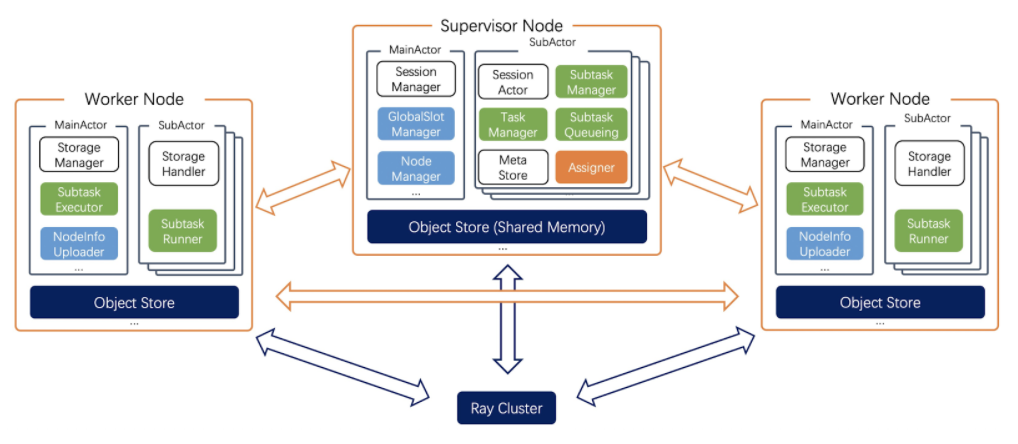• Ray actors implement Mars components such as the supervisor and the worker nodes, and use Ray remote calls for communication between distributed components of Mars. This allows Mars to focus only on the development of framework logic, instead of the complicated underlying details of distributed systems such as component communication, data serialization, and deployment.

• All data plane communications are based on the Ray object store. Mars puts computation results in the Ray object store, and data is exchanged between nodes through the Ray object store. Ray’s object store provides shared memory, automatic data GC, and data swapping between memory and external storage to help Mars efficiently manage large amounts of data across a large cluster.

• All Mars workers use Ray actor failover for automatic recovery. Mars only needs to add a configuration for fault recovery, without needing to care about the underlying monitoring, restart, and other issues.

• Fast and adaptive scale-out. Thanks to Ray’s actor model, there is no need to pull images first and then create a Mars cluster in a Ray cluster. In result, Mars clusters can scale up in less than 5 seconds when the workload increases.

• Fast and adaptive scale-in. Ray's object store is a global service (similar to the external shuffle service of a traditional batch computing engine). Therefore, the Mars worker does not need to migrate data when being offline, and can be offline in seconds.

While implementing the Ray backend, we also contributed auto scaling, scheduling, failover, operators, and stability optimizations to Mars.

XGBoost on Ray is a Ray based XGBoost distributed backend. Compared with the native XGBoost, it supports the following features:

• Multi-node and multi-GPU parallel training. The XGBoost backend supports automatic cross-GPU communication using NCCL2. You only need to start an actor on each GPU. For example, if you have two machines with four GPU cards, you can create eight actors by setting `gpus_per_actor = 1`. By doing this, each actor will hold a GPU card.

• Seamless integration with the popular distributed hyper-parameter tuning library Ray Tune.You only need to put the training code into a function, and then pass the function and the super-parameters of the tuning `tune.run`. You can perform parallel training and parameter search. At the same time, XGBoost on Ray also checks whether Ray Tune is used for parameter search, adjusts the XGBoost actor deployment policy, and then reports the results to Ray Tune.

• Supports loading distributed DataFrame as input data and distributed data. Supports Numpy/Pandas/Ray Datasets and other datasets. For non-distributed datasets, data is centrally loaded and sharded on the head node, and then written to the Ray object store for actor reading on each node. For distributed datasets, each node reads data directly into the Ray object store.

Generally, the time-consuming part of machine learning is data processing and training. Traditional solutions require external storage (usually distributed file systems) to exchange data between data processing and training, which is inefficient. In Mars and XGBoost On Ray, data is stored in the distributed object store provided by Ray, and exchanged through the shared memory object store (zero-copy) to avoid extra overhead. This results in higher efficiency.

In order to achieve highly efficient data exchange between Mars and XGBoost, we use Ray’s distributed dataset abstraction, Ray Datasets. Ray Datasets is a distributed Arrow dataset on Ray, which provides a unified data abstraction on Ray and serves as a standard method for loading data into a Ray cluster in parallel, doing basic parallel data processing, and efficiently exchanging data between Ray-based frameworks, libraries, and applications.

We support conversions between Mars DataFrame and Ray Datasets. XGBoost on Ray can use Mars DataFrame as input data for training and prediction.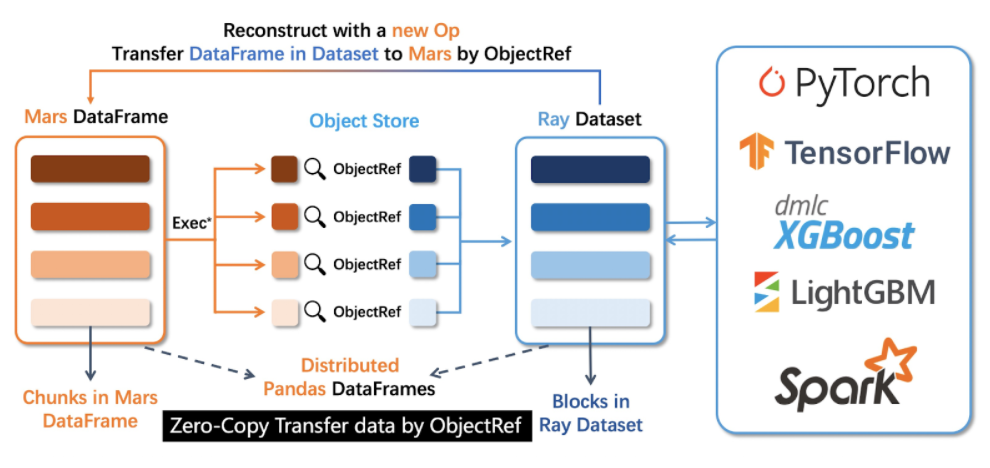Currently, objects stored in Mars are in Pandas format, so converting to Arrow format may incur some overhead. To resolve this, we’ve added support for zero-copy loading of both Arrow and Pandas data formats, which will be available in the next Ray release.

## LinkBuild a ML pipeline using Mars and XGBoost On Ray

This section of the post goes over an end-to-end code example based on generated data. The entire process consists of data preparation, model training, and model prediction. In a real scenario, data cleansing and feature engineering are also included.

The code below uses `make_classification` from Mars learn, a distributed version of scikit-learn provided by Mars, to generate a classification dataset.

``````1
2
3
4
import mars.dataframe as md
from mars.learn.datasets import make_classification
# generate data
X: md.DataFrame, y: md.DataFrame = make_classification(n_samples=n_samples, n_features=n_features, n_classes=n_classes, n_informative=n_informative, n_redundant=n_redundant, random_state=shuffle_seed)
``````

Like pandas, the `head` command can be used  to explore the first five lines of data: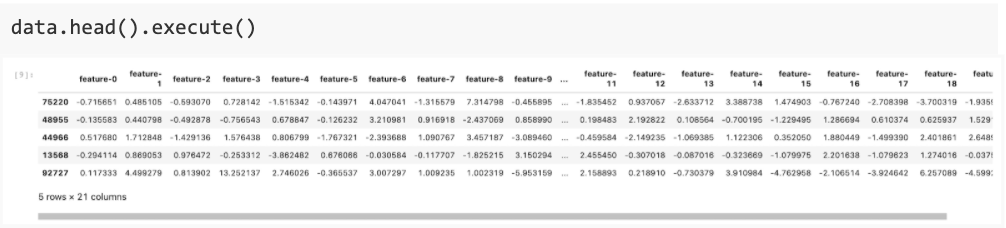`describe` can be used to view the data distribution: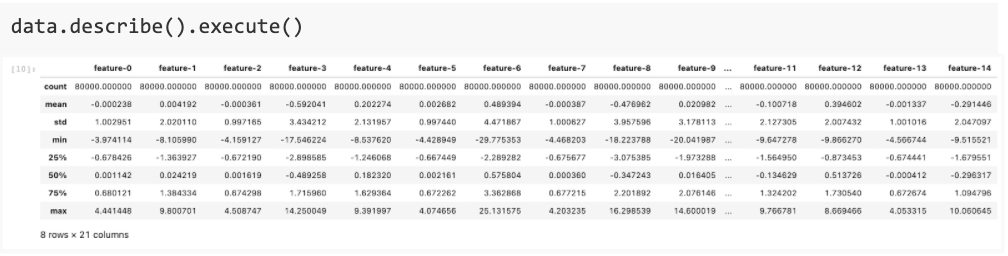You can also explore feature correlation. If highly correlated features appear, dimension reduction might be required: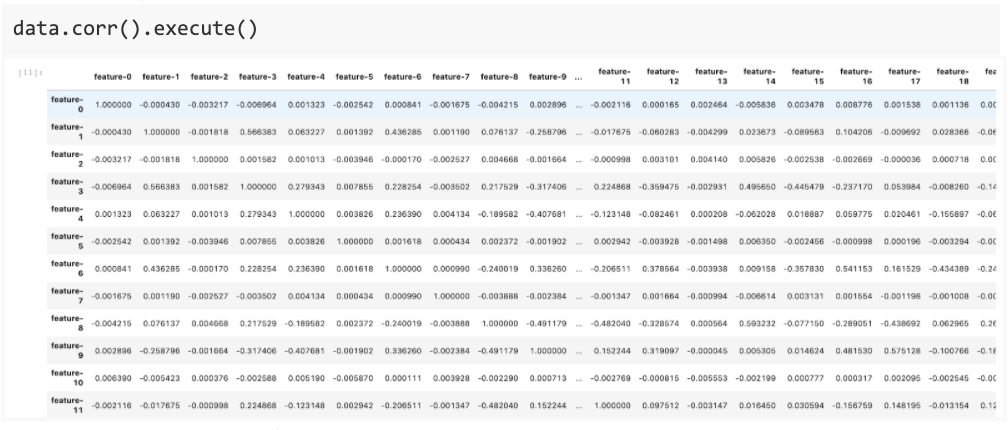The correlation between features can also be analyzed more intuitively through a plot: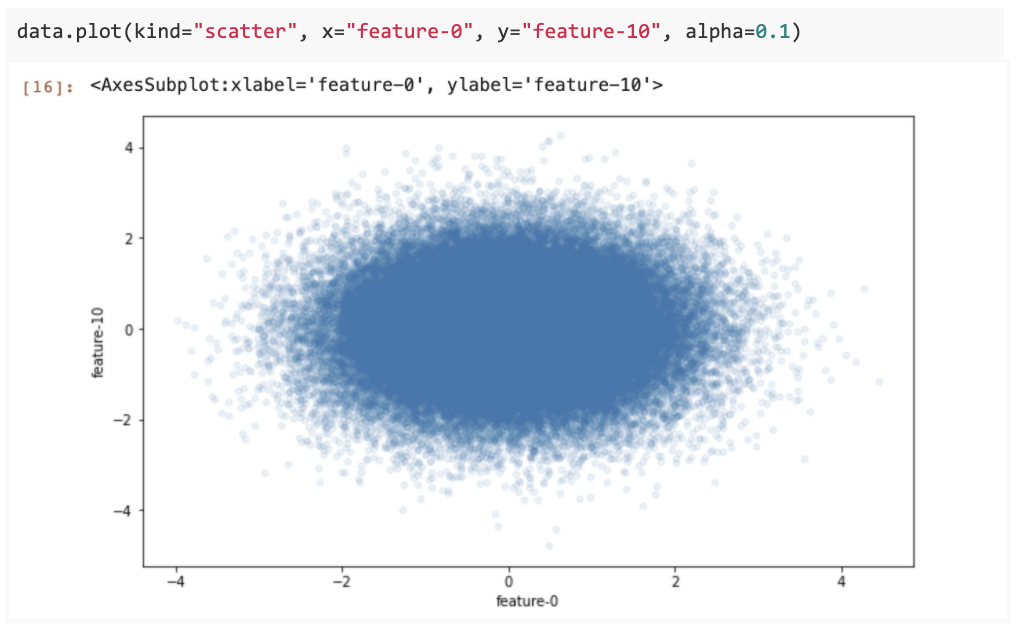Next, split the data into training sets and test sets.

``````1
2
3
from mars.learn.model_selection import train_test_split
X_train, X_test, y_train, y_test = train_test_split(
X, y, test_size=test_size, random_state=shuffle_seed)
``````

The next step is to create an XGBoost matrix for training and testing:

``````1
2
3
4
5
6
7
8
from xgboost_ray import RayDMatrix, RayParams, train, predict

# convert mars DataFrame to Ray dataset
ds_train = md.to_ray_dataset(df_train, num_shards=num_shards)
ds_test = md.to_ray_dataset(df_test, num_shards=num_shards)
# convert Ray dataset to RayDMatrix
train_set = RayDMatrix(data=ds_train, label="labels")
test_set = RayDMatrix(data=ds_test, label="labels")``````

The training process below uses the native XGBoost API for training. Note that the scikit-learn API provided by XGBoost-Ray could also be used for training. In a realistic training case, cross-validation or a separate validation set would be used to evaluate the model. In this case, these steps are omitted.

``````1
2
3
4
5
6
7
8
9
10
11
12
13
14
15
16
17
18
19
20
from xgboost_ray import RayDMatrix, RayParams, train, predict

evals_result = {}
params = {
'objective': 'multi:softmax',
'eval_metric': ['mlogloss', 'merror'],
'num_class': n_classes,
'eta': 0.1,
'seed': 42
}
bst = train(
params=params,
dtrain=train_set,
num_boost_round=200,
evals=[(train_set, 'train')],
evals_result=evals_result,
verbose_eval=100,
ray_params=ray_params
)
``````

The scikit-learn style API could also have been used:

``````1
2
3
4
5
6
7
8
9
10
from xgboost_ray import RayXGBClassifier

clf = RayXGBClassifier(
n_jobs=4,  # In XGBoost-Ray, n_jobs sets the number of actors
random_state=42
)
# scikit-learn API will automatically convert the data
# to RayDMatrix format as needed.
# You can also pass X as a RayDMatrix, in which case y will be ignored.
clf.fit(train_set)
``````

The overall prediction process is similar to that of training.

``````1
2
3
4
5
6
from xgboost_ray import RayParams, predict

# predict on a test set.
pred = predict(bst, test_set, ray_params=ray_params)
precision = (ds_test.dataframe['labels'].to_pandas() == pred).astype(int).sum() / ds_test.dataframe.shape
print(f"Prediction Accuracy: {precision}")``````

If the model is trained by the scikit-learn style API, that API should be used for prediction:

``````1
2
3
4
5
pred_ray = clf.predict(test_set)
print(pred_ray)

pred_proba_ray = clf.predict_proba(test_set)
print(pred_proba_ray)``````

The code below shows it is possible to build a complete end-to-end distributed data processing and training job in less than 100 lines.

``````1
2
3
4
5
6
7
8
9
10
11
12
13
14
15
16
17
18
19
20
21
22
23
24
25
26
27
28
29
30
31
32
33
34
35
36
37
38
39
40
41
42
43
44
45
46
47
48
49
50
51
52
53
54
55
56
57
58
59
60
61
62
63
64
65
66
67
68
import logging
import ray
import numpy as np
import mars.dataframe as md
from mars.learn.model_selection import train_test_split
from mars.learn.datasets import make_classification
from xgboost_ray import RayDMatrix, RayParams, train, predict

logger = logging.getLogger(__name__)
logging.basicConfig(format=ray.ray_constants.LOGGER_FORMAT, level=logging.INFO)

n_features:int,
n_classes: int,
test_size: float = 0.1,
shuffle_seed: int = 42):
n_informative = int(n_features * 0.5)
n_redundant = int(n_features * 0.2)
# generate dataset
X, y = make_classification(n_samples=n_samples, n_features=n_features,
n_classes=n_classes, n_informative=n_informative,
n_redundant=n_redundant, random_state=shuffle_seed)
X, y = md.DataFrame(X), md.DataFrame({"labels": y})
X.columns = ['feature-' + str(i) for i in range(n_features)]
# split dataset
X_train, X_test, y_train, y_test = train_test_split(
X, y, test_size=test_size, random_state=shuffle_seed)
return md.concat([X_train, y_train], axis=1), md.concat([X_test, y_test], axis=1)

def main(*args):
n_samples, n_features, worker_num, worker_cpu, num_shards = 10 ** 5, 20, 10, 8, 10
ray_params = RayParams(
num_actors=10,
cpus_per_actor=8
)

# setup mars
mars.new_ray_session(worker_num=worker_num, worker_cpu=worker_cpu, worker_mem=8 * 1024 ** 3))
n_classes = 10
df_train, df_test = _load_data(n_samples, n_features, n_classes, test_size=0.2)
# convert mars DataFrame to Ray dataset
ds_train = md.to_ray_dataset(df_train, num_shards=num_shards)
ds_test = md.to_ray_dataset(df_test, num_shards=num_shards)
train_set = RayDMatrix(data=ds_train, label="labels")
test_set = RayDMatrix(data=ds_test, label="labels")

evals_result = {}
params = {
'objective': 'multi:softmax',
'eval_metric': ['mlogloss', 'merror'],
'num_class': n_classes,
'eta': 0.1,
'seed': 42
}
bst = train(
params=params,
dtrain=train_set,
num_boost_round=200,
evals=[(train_set, 'train')],
evals_result=evals_result,
verbose_eval=100,
ray_params=ray_params
)
# predict on a test set.
pred = predict(bst, test_set, ray_params=ray_params)
precision = (ds_test.dataframe['labels'].to_pandas() == pred).astype(int).sum() / ds_test.dataframe.shape
logger.info("Prediction Accuracy: %.4f", precision)``````

Currently, Mars on Ray is widely used at Ant Group and in the open source Mars Community. We plan to further optimize Mars and Ray in the following ways:

• Mars & XGBoost worker collocation. Currently, both Mars and XGBoost on Ray use Ray’s placement group separately for custom scheduling. Generally, Mars & XGBoost workers are not located on the same nodes. When XGBoost reads Ray Datasets data and converts it to the internal format of the DMatrix, it needs to pull data from the object store of other nodes, which may incur data transfer overhead. In the future, Mars and XGBoost workers will be collocated on the same nodes to reduce data transfer overhead and speed up training.

• Task Scheduling optimization. Currently, we are working on locality aware scheduling. This mechanism will allow scheduling computation tasks to the nodes where the data lives and reduce the overhead from internal data transfer. This effort is partially done in some scenarios and needs more optimizations.

• DataFrame storage format optimization. When a Pandas DataFrame is stored in the object store, the entire Pandas DataFrame is serialized and then written into the object store. When manipulating partial columns of a DataFrame object, the entire DataFrame object needs to be rewritten into another Ray object, resulting in additional memory copy and memory usage overhead.  We plan to store Pandas DataFrames and Arrow tables on a column-by-column basis to avoid this overhead.

Pandas originated from financial companies. A large number of businesses at Ant Group also use Pandas for data analysis and processing. We believe that with the optimization of Mars and Ray, we can broaden use cases, and further speed up the productivity of data and algorithm engineers.